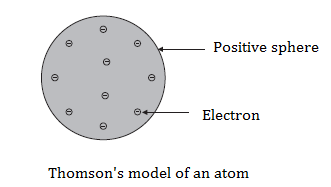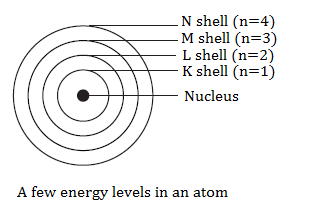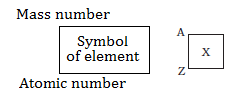# CBSE Class 9 Science Chapter 4 Structure Of The Atom Notes

Atoms are the smallest particle of a chemical element that can possibly exist. We have learnt that atoms and molecules are the basic building blocks of matter and the existence of different kinds of matter is due to different atoms which constitute them. Through CBSE class 9 science chapter 4 notes for Structure of atoms we understand the structure and composition of atoms in detail.

Chapter 4 Structure Of The Atom Notes:-

Topics covered in Structure Of Atom Chapter:

• Charged Particles In Matter
• The Structure Of An Atom
• How are Electrons Distributed in Different Orbits (Shells)?
• Valency
• Atomic Number and Mass Number
• Isotopes

### Charged Particles In Matter

• The discovery of electron and proton is credited to J.J. Thomson and E.Goldstein, respectively.
• J.J. Thomson proposed that electrons are embedded in a positive sphere
• Electron was represented as ‘e-‘ and proton as ‘p+’. The mass of a proton is taken as one unit and its charge as plus one where the mass of an electron was considered to be negligible and its charge minus one
• It seemed that atom consisted of electrons and protons which balanced their charges mutually

### Structure Of Atom

• Dalton’s atomic theory suggested that the atom was indivisible and indestructible. However, the discovery of two fundamental particles in the atom the electrons and protons led to the failure of this aspect of the theory
• Hence, J.J. Thomson was the first to propose a model for the structure of an atom

#### Thomson’s Model Of An Atom

• Thomson proposed a model of an atomHe proposed the following:

• An atom consists of a positively charged sphere and the electrons are embedded in it.
• The negative and positive charges are equal in magnitude. So, the atom as a whole is electrically neutral.

#### Rutherford’s Model Of An Atom

Rutherford carried out an experiment where fast-moving alpha particles were made to fall on a thin gold foil. The following were his observations:

• Most of the fast moving a-particles passed straight through the gold foil.
• Some of the a-particles were deflected by the foil by small angles.
• Surprisingly one out of every 12000 particles appeared to rebound.

Following were his conclusions from the a-particle scattering experiment:

• Most of the space inside the atom is empty because most of the a-particles passed through the gold foil without getting deflected.
• Very few particles were deflected from their path, indicating that the positive charge of the atom occupies very little space.
• A very small fraction of a-particles were deflected by 1800, indicating that all the positive charge and mass of the gold atom were concentrated in a very small volume within the atom.

On the basis of his experiment, Rutherford put forward the nuclear model of an atom, which had the following features:

• There is a positively charged centre in an atom called the nucleus. Nearly all the mass of an atom resides in the nucleus.
• The electrons revolve around the nucleus in circular paths.
• The size of the nucleus is very small as compared to the size of the atom.

Drawbacks of Rutherford’s model of the atom:

• Was unable to explain the stability of an atom
• The theory was incomplete as it did not mention anything about the arrangement of electrons in the orbit
• Failed to explain line spectra of an atom

#### Bohr’s Model Of Atom

Neils Bohr put forward the following postulates about the model of an atom:

• Only certain special orbits known as discrete orbits of electrons are allowed inside the atom.
• While revolving in discrete orbits the electrons do not radiate energy.#### Neutrons

Neutrons have no charges, they have a mass nearly equal to that of a proton. They are found in nucleus of all atoms except in hydrogen. They are represented as ‘n’. Hence, the mass of an atom is given by the sum of masses of protons and neutrons present in the nucleus.

#### Distribution Of Electrons In Different Orbits

The distribution of electrons into different orbits of an atom was suggested by Bohr and Bury.

The following rules are followed for writing the number of electrons in different energy levels or shells:

• The maximum number of electrons present in a shell is given by the formula 2n2, where ‘n’ is the orbit number or energy level index, 1,2,3,….

Hence the maximum number of electrons in different shells are as follows:

first orbit or K-shell will be = 2 × 12 = 2,

second orbit or L-shell will be = 2 × 22 = 8,

third orbit or M-shell will be = 2 × 32 = 18,

fourth orbit or N-shell will be = 2 × 42 = 32, and so on.

• The maximum number of electrons that can be accommodated in the outermost orbit is 8.
• Electrons are not accommodated in a given shell unless the inner shells are filled. That is, the shells are filled in a step-wise manner.

#### Atomic Number And Mass Number

Atomic number – denoted by ‘Z’. It is defined as the total number of protons present in the nucleus of an atom.

Mass Number – denoted by ‘A’. Protons and neutrons are also called nucleons. Mass number is defined as the sum total number of protons and neutrons present in the nucleus of an atom.

In the notation for an atom, the atomic number, mass number and symbol of the element are to be written as:#### Isotopes

It can be defined as the atoms of the same element, having the same atomic number but different mass numbers. Therefore, we can say that there are three isotopes of a hydrogen atom, namely protium, deuterium and tritium.

Applications of Isotopes:

• An isotope of iodine is used in the treatment of goitre.
• An isotope of uranium is used as a fuel in nuclear reactors.
• An isotope of cobalt is used in the treatment of cancer.

#### Isobars

It can be defined as the atoms of different elements with different atomic numbers, which

have the same mass number. Example – calcium, atomic number 20, and argon, atomic

number 18. The number of protons in these atoms is different, but the mass number of

both these elements is 40

Explore more about CBSE class 9 chapter 4 notes here at BYJU’S.

 NCERT Related Articles NCERT Solutions for Class 12 NCERT Solutions for Class 11 NCERT Solutions for Class 10 NCERT Solutions for Class 12 Maths NCERT Solutions for Class 11 Maths NCERT Solutions for Class 10 Maths NCERT Solutions for Class 12 Physics NCERT Solutions for Class 11 Physics NCERT Solutions for Class 10 Science NCERT Solutions for Class 12 Chemistry NCERT Solutions for Class 11 Chemistry NCERT Solutions for Class 6 NCERT Solutions for Class 12 Biology NCERT Solutions for Class 11 Biology NCERT Solutions for Class 6 Maths NCERT Solutions for Class 9 NCERT Solutions for Class 8 NCERT Solutions for Class 6 Science NCERT Solutions for Class 9 Maths NCERT Solutions for Class 8 Maths NCERT Solutions for Class 4 NCERT Solutions for Class 9 Science NCERT Solutions for Class 8 Science NCERT Solutions for Class 4 Maths NCERT Solutions for Class 5 NCERT Solutions for Class 7 NCERT Solutions for Class 7 Maths NCERT Solutions for Class 4 Science NCERT Solutions for Class 5 Maths NCERT Solutions for Class 7 Science NCERT Solutions NCERT Books NCERT Solutions for Class 5 Science